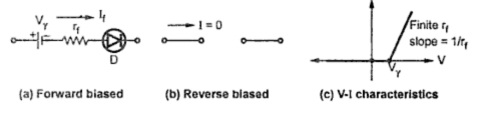### Circuit Models of a Diode

The diode is required to be replaced by the equivalent circuit in many practical electronic circuits, for the analysis purpose. Such an equivalent circuit of a diode is a circuit model of a diode. There are three methods of replacing diode by its circuit model, which are,
1. Practical diode model
2. Ideal diode model
3. Piecewise linear model
1.1 Practical diode model
We have seen that in forward biased diode, the total voltage drop across the diode is Vwhich consists of drop due to barrier potential which is almost equal to cut-in voltage Vγ and the drop across the internal forward dynamic resistance rf of the diode. While when reverse biased, reverse saturation current is very small and practically neglected. Hence reverse biased diode is practically assumed to be open circuit.
Thus the practical diode model consists of a battery equal to cut-in voltage and the forward resistance, in series with an ideal diode, in forward biased condition. The Fig. 1(a) shows this model. In reverse biased, it is open circuited. The Fig. 1(b) shows this model. While the Fig. 1(c) shows the corresponding V-I characteristics.Fig. 1 Practical diode model
Note : An external circuit to the diode must be designed properly so as to control the forward current through the diode.
1.2 Ideal Diode Model
In many cases, as the forward resistance of diode is small and cut-in voltage is also small, the diode is assumed to be an ideal diode.
In case of ideal diode, it is assumed that it starts conducting instantaneously when applied voltage is just greater than zero and the drop across the conducting diode is zero. So conducting diode can be ideally replaced by a short circuit, for the analysis of various diode circuits. The Fig. 2 shows the ideal diode characteristics.Fig. 2  Ideal diode model
1.3 Piecewise Linear Model of Diode
Another way to analyse the diode circuits is to approximate the V-I characteristics of a diode using only straight line i.e. linear relationships.
In such approximation, the diode forward resistance is neglected and the diode is assumed to conduct instantaneously when applied forward biased voltage VD is equal to cut-in voltage Vγ. And then it is assumed that current increases instantaneously giving straight line nature of V-I characteristics. While in reverse biased condition whenVD < 0, the diode does not conduct at all.
Hence when diode forward resistance is assumed zero, the circuit model of diode is as shown in the Fig. 3(a). In reverse biased, the diode is open circuit as shown in the Fig. 3(b). As the diode conducts at VD = Vγ, the V-I characteristics with straight lines is as shown in the Fig. 3(c).
As the method models the diode with the pieces of straight lines, the name given to such approximation is piecewise-linear method. The characteristics of diode shown in the Fig. 3(c) are called the piecewise linear diode characteristics.Fig. 3  Linear piecewise model of diode with rf  = 0 Ω
Finite rf : In the above discussion, rf is neglected as very small. But if rf is considered to be finite, then in V-I characteristics, forward biased characteristics is a straight line with a slope equal to reciprocal of rf. In reverse biased the diode is still assumed to be open circuited. The linear piecewise model with finite rf is shown in the Fig. 4.Fig. 4  Linear piecewise model of diode with finite rf
The diode equivalent models are summarized in the table 1.Table 1.  Diode equivalent circuits
In all the above models, the diode is assumed to be open circuit, when reverse biased. The value of Vγ = 0.2 V for germanium and 0.7 V for silicon diodes.
1.4 Analysis oF Diode Circuits
Using the appropriate diode model, the circuit consisting of many diodes, resistance and sources can be analysed. The analysis starts by assuming certain diodes ON and other diodes OFF. This assumption is based on circuit inspection and polarities of sources connected in the circuit. Then ON diodes must be replaced by a battery of voltage Vγ and a series resistance rf. rf if is not known, it can be assumed zero. The OFF diodes must be replaced by open circuits. Then the circuit becomes linear and can be analysed using Kirchhoff's laws. The assumption of ON diodes can be verified from the directions of currents obtained by Kirchhoff's laws analysis. If the direction of current obtained for a particular diode is reverse i.e. from n to p for a diode, the assumption that diode is ON is incorrect. In such a case, ON diode must be replaced by OFF and entire analysis of the circuit must be done once again. The OFF state assumption of diodes can be verified by calculating voltages across open circuits, used to replace OFF diodes. If we get polarity of any such voltage in forward direction, with magnitude greater than Vγ, the assumption of OFF diode is incorrect. In such a case, that diode must be replaced by ON state model, and once again entire analysis must be done. If the voltages are in reverse direction or in forward direction with magnitude less than  Vγ, the assumptions of OFF diodes get justified. This method is used to analyse various complex diode circuits.
Note : Thus by using model of a diode, the mathematical analysis of the circuit can be done, which helps us to predict the circuit performance before actually building and testing the diode circuit in the laboratory.